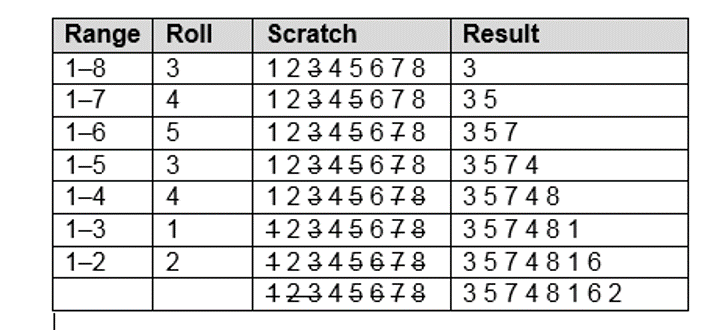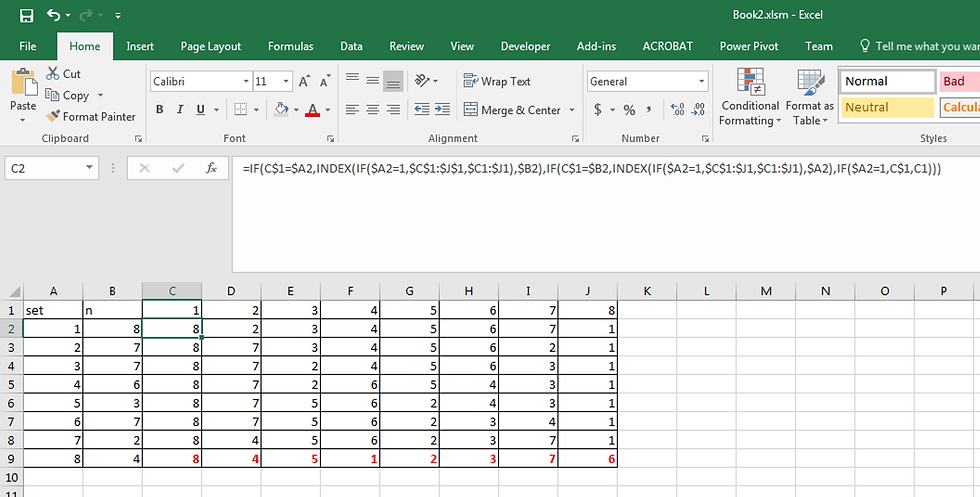top of pageSearch

# Fisher Yates Shuffle

When generating random samples, Relativity uses an algorithm called the Fisher-Yates shuffle. See the documentation of the shuffling process on the kCura website here. The Fisher-Yates shuffle creates a random sequence of items in a closed set by picking a random number, n, and then removing the nth occurrence of that number (counting from the end) in the original set and moving it to a new list. This process is repeated until all of the numbers in the set have been removed from the original set. See this example from the Wikipedia page for the Fisher-Yates algorithm:So after the first selection, we pick a random number N between 1 and 7 (we 'roll' the dice so to speak), 1 to 7 being the count of the remaining number of items in the set, and then take out the nth occurrence of that number counting from the end of the list.

Random sampling can be performed in Excel using the method described in this blog post. In this example we put the numbers in our set from 1 to 8 in column A. Then we use this formula:

=RANDBETWEEN(\$A\$2,\$A\$9)

to generate random selections from the set in column B. But the trouble is that the numbers repeat. Proceed by entering the numbers from the set in first row beginning in column C. Then enter this formula in C2:

=IF(C\$1=\$A2,INDEX(IF(\$A2=1,\$C\$1:\$J\$1,\$C1:\$J1),\$B2),IF(C\$1=\$B2,INDEX(IF(\$A2=1,\$C\$1:\$J1,\$C1:\$J1),\$A2),IF(\$A2=1,C\$1,C1)))

. . . then drag it to the right and down. The numbers in red at C3:J9 are our random set of numbers.bottom of page